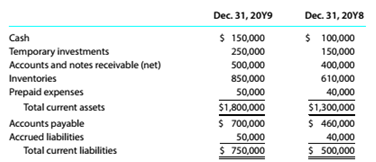Chapter 9, Problem 9.6E

Chapter
Section
Textbook Problem

Current position analysis The following data were taken from the comparative balance sheet of Oshorn Sisters Company for the years ended December 31. 20Y9 and December 31. 20Y8:a. Determine for each year (1) the working capital, (2) the current ratio, and (3) the quick ratio. b. What conclusions can be drawn from these data?

To determine

Concept Introduction:

Working Capital: It is the difference between the current assets and the current liabilities of the business. Working capital is the amount which is used by businesses to meet its short-term expenses.

Current Ratio: Current ratio is the ratio of current assets with current liabilities, it tells about the capability of a company to handle daily expenses. Best current ratio is 2:1.

Quick Ratio: Quick ratio is the ratio of quick assets with current liabilities it tells about the liquidity of the company/ business. Best possible quick ratio is 1:1.

Requirement-1:

To Calculate:

Working capital, current ratio and quick ratio.

Explanation
 Particulars 2019 2018 Current Assets(A) 1800000 1300000 Current Liabilities (B) 750000 500000 Inventory (C) 850000 610000 Prepaid Expenses(D)
To determine

Concept Introduction:

Working Capital: It is the difference between the current assets and the current liabilities of the business. Working capital is the amount which is used by the business to meet its short term expenses.

Current Ratio: Current ratio is the ratio of current assets with current liabilities it tells about the how much company strong to meet its day to day expenses. Best current ratio is 2:1.

Quick Ratio: Quick ratio is the ratio of quick assets with current liabilities it tells about the liquidity of the company/ business. Best possible quick ratio is 1:1.

Requirement-2:

To Indicate:

What Conclusion from above ratios?

Still sussing out bartleby?

Check out a sample textbook solution.

See a sample solution

The Solution to Your Study Problems

Bartleby provides explanations to thousands of textbook problems written by our experts, many with advanced degrees!

Get Started